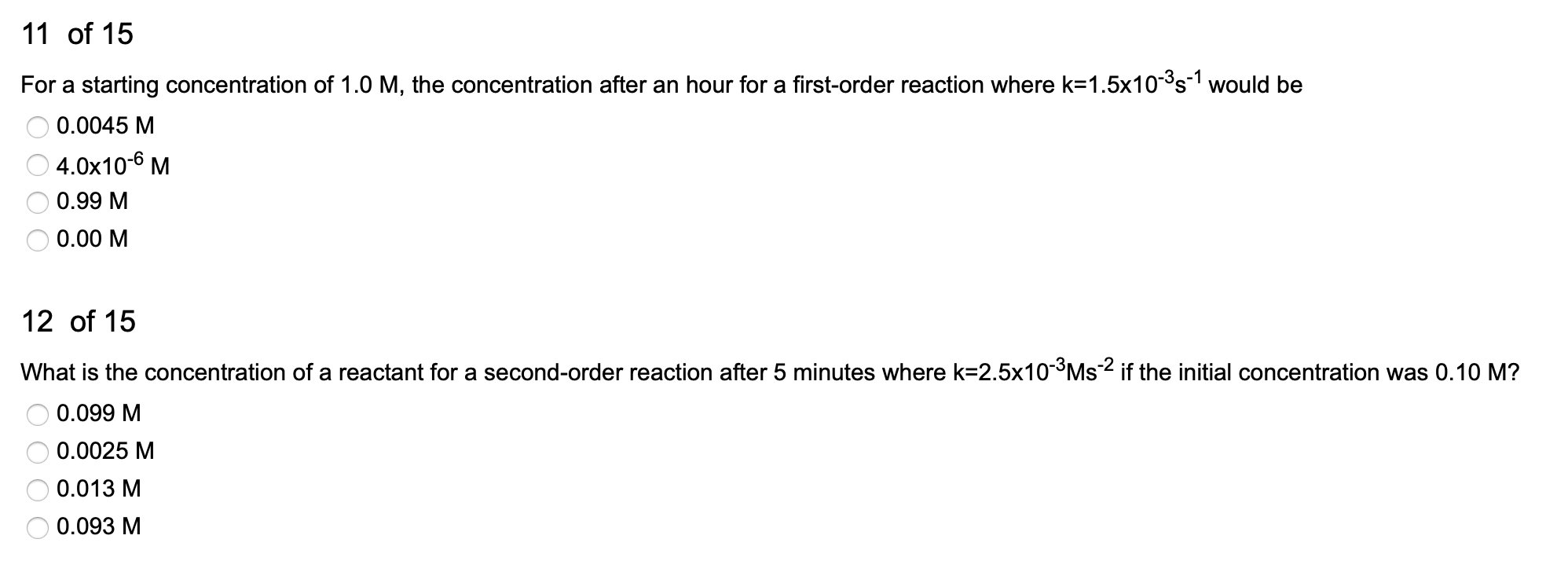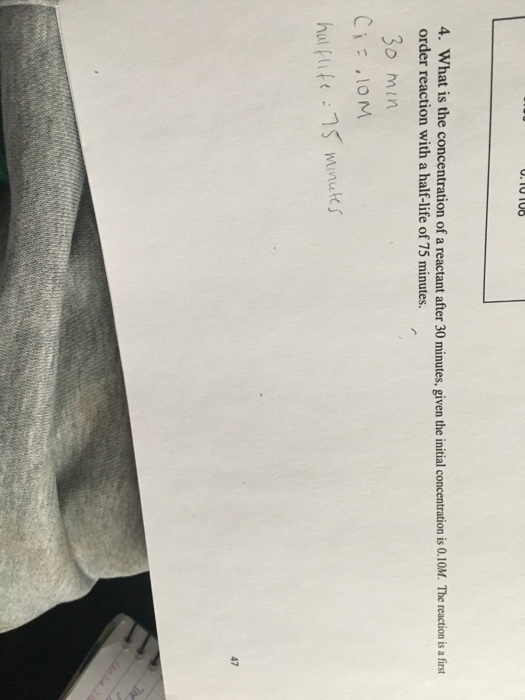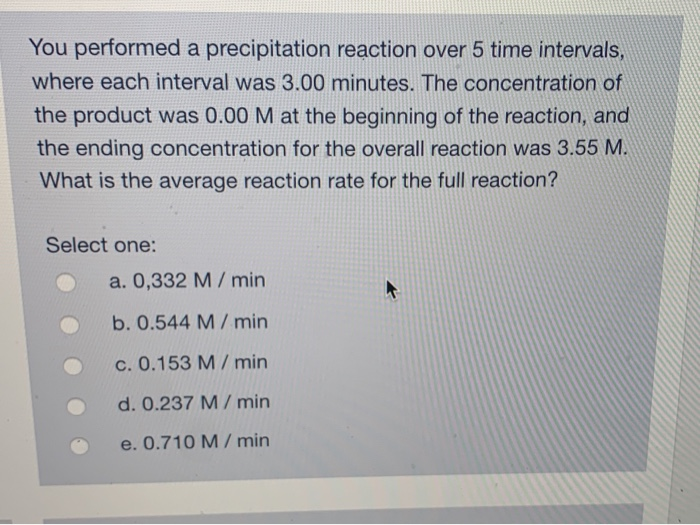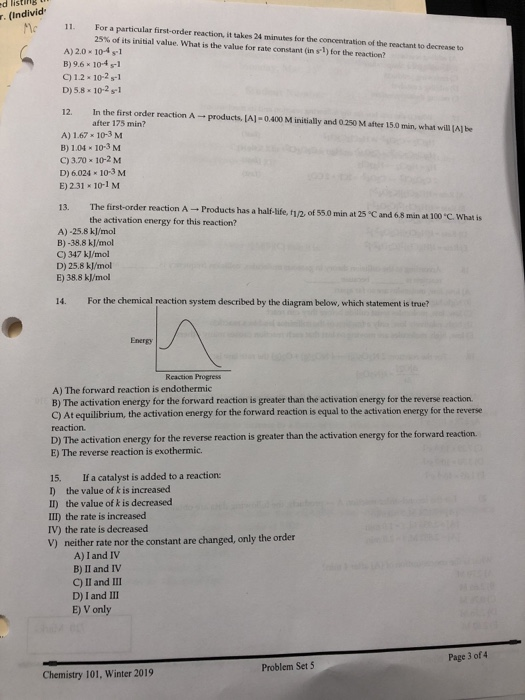Question

# What is the concentration of A for a 1st order reaction after 3.00 minutes where the...

What is the concentration of A for a 1st order reaction after 3.00 minutes where the initial concentration of A is 0.614 M and k = 0.000165/s.

A 0.982 M

B 0.613 M

C 0.596 M

D 0.573 M

We need at least 10 more requests to produce the answer.

0 / 10 have requested this problem solution

The more requests, the faster the answer.

All students who have requested the answer will be notified once they are available.

#### Earn Coins

Coins can be redeemed for fabulous gifts.

Similar Homework Help Questions
• ### What is the concentration of A after 50.5 minutes for the second order reaction A →...

What is the concentration of A after 50.5 minutes for the second order reaction A → Products when the initial concentration of A is 0.250 M? (k = 0.117 M⁻¹min⁻¹)

• ### For a 1st order reaction the logarithm of concentration is linear to time. The linear equation...

For a 1st order reaction the logarithm of concentration is linear to time. The linear equation of the following curve is lnC = lnC0 – 0.2t; where C is the molar concentration (M) of a reactant, C0 is the molar concentration at time t = 0 (the starting concentration or the initial concentration), and in this particular case C0 = 10 M; and t is time in seconds. What is the slope (and the unit) of the curve? What will...

• ### 11 of 15 For a starting concentration of 1.0 M, the concentration after an hour for...11 of 15 For a starting concentration of 1.0 M, the concentration after an hour for a first-order reaction where k=1.5x10-35-1 would be O 0.0045 M 04.0x10-6 M O 0.99 M O 0.00 M 12 of 15 What is the concentration of a reactant for a second-order reaction after 5 minutes where k=2.5x10-3Ms-2 if the initial concentration was 0.10 M? O 0.099 M O 0.0025 M O 0.013 M O 0.093 M

• ### What is the concentration of a reactant after 30 minutes, given the initial concentration is 0.10M....What is the concentration of a reactant after 30 minutes, given the initial concentration is 0.10M. The reaction is a first order reaction with a half-life of 75 minutes.

• ### You performed a precipitation reaction over 5 time intervals where each interval was 3.00 minutes. The concentratio...You performed a precipitation reaction over 5 time intervals where each interval was 3.00 minutes. The concentration of the product was 0.00 M at the beginning of the reaction, and the ending concentration for the overall reaction was 3.55 M. What is the average reaction rate for the full reaction? Select one: a. 0,332 M/ min b.0.544 M/ min c. 0.153 M/ min min d. 0.237 M/ min e. 0.710 M/

• ### d listing r. (Individ For a particular first-order reaction, it takes 24 minutes for the concentration...d listing r. (Individ For a particular first-order reaction, it takes 24 minutes for the concentration of the reactant to decrease to 25% of its initial value, what is the value for rate constant (in S-1) for the reaction? A) 2.0-104 s-1 B)9.6 x 10-4 s-1 C) 1.2 × 10-2 s-1 D)5.8 102s-1 12. In the first order reaction A → products, [A1-0.400 M initially and 0250 M after 15.0 mun, what willl after 175 min? e A) 1.67-10-3 M...

• ### A zero-order reaction has a constant rate of 2.90×10−4 M/s. If after 35.0 seconds the concentration...

A zero-order reaction has a constant rate of 2.90×10−4 M/s. If after 35.0 seconds the concentration has dropped to 3.00×10−2 M, what was the initial concentration?

• ### For a zero order reaction, the initial reactant concentration is 0.84 M and after 26 s...

For a zero order reaction, the initial reactant concentration is 0.84 M and after 26 s the concentration is 0.68M. Approximately how many seconds after the start of the reaction does it take for the reactant concentration to decrease to 0.21 M? a. 40s b. 603s c. 102s d. 80s e. 120s Please explain what concept/equation used to get the answer.

• ### 1. The rate constant for a certain reaction is k=3.70x10-3 s-1. If the initial reactant concentration...

1. The rate constant for a certain reaction is k=3.70x10-3 s-1. If the initial reactant concentration was 0.400 M, what will the concentration be after 3.00 minutes? 2. A zero-orderreaction has a constant rate of 3.30x10-4 M/s. If after 80.0 seconds the concentration has dropped to 8.00x20-2 M, what was the initial concentration?

• ### Consider the reaction A ⟶ Products. The rate law for this reaction is rate = k[A]...

Consider the reaction A ⟶ Products. The rate law for this reaction is rate = k[A] where k= 3.00 X × 10-3 s-1 at a particular temperature. If the initial [A] = 0.500 M, what will be the concentration of A after 2.00 minutes